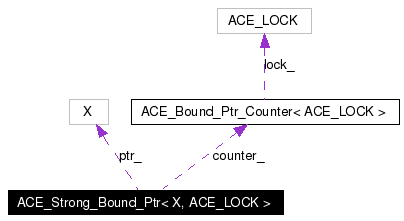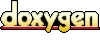# ACE_Strong_Bound_Ptr< X, ACE_LOCK > Class Template Reference

This class implements support for a reference counted pointer. More...

`#include <Bound_Ptr.h>`

Collaboration diagram for ACE_Strong_Bound_Ptr< X, ACE_LOCK >:[legend]
List of all members.

## Public Member Functions

ACE_Strong_Bound_Ptr (X *p=0)
ACE_Strong_Bound_Ptr (auto_ptr< X > p)
ACE_Strong_Bound_Ptr (const ACE_Strong_Bound_Ptr< X, ACE_LOCK > &r)
Copy constructor binds and to the same object.

ACE_Strong_Bound_Ptr (const ACE_Weak_Bound_Ptr< X, ACE_LOCK > &r)
Constructor binds and to the same object.

~ACE_Strong_Bound_Ptr (void)
Destructor.

void operator= (const ACE_Strong_Bound_Ptr< X, ACE_LOCK > &r)
Assignment operator that binds and to the same object.

void operator= (const ACE_Weak_Bound_Ptr< X, ACE_LOCK > &r)
Assignment operator that binds and to the same object.

bool operator== (const ACE_Strong_Bound_Ptr< X, ACE_LOCK > &r) const
bool operator== (const ACE_Weak_Bound_Ptr< X, ACE_LOCK > &r) const
bool operator== (X *p) const
bool operator!= (const ACE_Strong_Bound_Ptr< X, ACE_LOCK > &r) const
Inequality operator, which is the opposite of equality.

bool operator!= (const ACE_Weak_Bound_Ptr< X, ACE_LOCK > &r) const
Inequality operator, which is the opposite of equality.

bool operator!= (X *p) const
Inequality operator, which is the opposite of equality.

X * operator-> (void) const
Redirection operator.

X & operator * (void) const
Dereference operator.

X * get (void) const
Get the pointer value.

void reset (X *p=0)
void reset (auto_ptr< X > p)
int null (void) const

## Public Attributes

ACE_ALLOC_HOOK_DECLARE
Declare the dynamic allocation hooks.

## Private Types

typedef ACE_Bound_Ptr_Counter<
ACE_LOCK >
COUNTER
The ACE_Bound_Ptr_Counter type.

## Private Attributes

COUNTERcounter_
The reference counter.

X * ptr_
The underlying object.

## Friends

class ACE_Weak_Bound_Ptr< X, ACE_LOCK >

## Detailed Description

### template<class X, class ACE_LOCK> class ACE_Strong_Bound_Ptr< X, ACE_LOCK >

This class implements support for a reference counted pointer.

Assigning or copying instances of an ACE_Strong_Bound_Ptr will automatically increment the reference count of the underlying object. When the last instance of an ACE_Strong_Bound_Ptr that references a particular object is destroyed or overwritten, it will invoke delete on its underlying pointer.

Definition at line 111 of file Bound_Ptr.h.

## Member Typedef Documentation

 template typedef ACE_Bound_Ptr_Counter ACE_Strong_Bound_Ptr< X, ACE_LOCK >::COUNTER` [private]`
 The ACE_Bound_Ptr_Counter type. Definition at line 198 of file Bound_Ptr.h.

## Constructor & Destructor Documentation

 template ACE_Strong_Bound_Ptr< X, ACE_LOCK >::ACE_Strong_Bound_Ptr ( X * p = 0 ) ` [inline, explicit]`
 Constructor that initializes an ACE_Strong_Bound_Ptr to point to the object

immediately. Definition at line 147 of file Bound_Ptr.inl. References ACE_Strong_Bound_Ptr< X, ACE_LOCK >::COUNTER. ```00148 : counter_ (COUNTER::create_strong ()), 00149 ptr_ (p) 00150 { 00151 } ```

 template ACE_Strong_Bound_Ptr< X, ACE_LOCK >::ACE_Strong_Bound_Ptr ( auto_ptr< X > p ) ` [inline, explicit]`
 Constructor that initializes an ACE_Strong_Bound_Ptr by stealing ownership of an object from an auto_ptr. Definition at line 154 of file Bound_Ptr.inl. References ACE_Strong_Bound_Ptr< X, ACE_LOCK >::COUNTER. ```00155 : counter_ (COUNTER::create_strong ()), 00156 ptr_ (p.release()) 00157 { 00158 } ```

 template ACE_Strong_Bound_Ptr< X, ACE_LOCK >::ACE_Strong_Bound_Ptr ( const ACE_Strong_Bound_Ptr< X, ACE_LOCK > & r ) ` [inline]`
 Copy constructor binds and to the same object. Definition at line 161 of file Bound_Ptr.inl. ```00162 : counter_ (r.counter_), 00163 ptr_ (r.ptr_) 00164 { 00165 COUNTER::attach_strong (this->counter_); 00166 } ```

 template ACE_Strong_Bound_Ptr< X, ACE_LOCK >::ACE_Strong_Bound_Ptr ( const ACE_Weak_Bound_Ptr< X, ACE_LOCK > & r ) ` [inline]`
 Constructor binds and to the same object. Definition at line 169 of file Bound_Ptr.inl. References ACE_Strong_Bound_Ptr< X, ACE_LOCK >::ptr_. ```00170 : counter_ (r.counter_), 00171 ptr_ (r.ptr_) 00172 { 00173 // When creating a strong pointer from a weak one we can't assume that the 00174 // underlying object still exists. Therefore we must check for a return value 00175 // of -1, which indicates that the object has been destroyed. 00176 if (COUNTER::attach_strong (this->counter_) == -1) 00177 { 00178 // Underlying object has already been deleted, so set this pointer to null. 00179 this->counter_ = COUNTER::create_strong (); 00180 this->ptr_ = 0; 00181 } 00182 } ```

 template ACE_Strong_Bound_Ptr< X, ACE_LOCK >::~ACE_Strong_Bound_Ptr ( void ) ` [inline]`
 Destructor. Definition at line 185 of file Bound_Ptr.inl. References ACE_Strong_Bound_Ptr< X, ACE_LOCK >::ptr_. ```00186 { 00187 if (COUNTER::detach_strong (this->counter_) == 0) 00188 delete this->ptr_; 00189 } ```

## Member Function Documentation

 template X * ACE_Strong_Bound_Ptr< X, ACE_LOCK >::get ( void ) const` [inline]`
 Get the pointer value. Definition at line 280 of file Bound_Ptr.inl. References ACE_Strong_Bound_Ptr< X, ACE_LOCK >::ptr_. ```00281 { 00282 return this->ptr_; 00283 } ```

 template int ACE_Strong_Bound_Ptr< X, ACE_LOCK >::null ( void ) const` [inline]`
 Allows us to check for NULL on all ACE_Strong_Bound_Ptr objects. Definition at line 286 of file Bound_Ptr.inl. References ACE_Strong_Bound_Ptr< X, ACE_LOCK >::ptr_. ```00287 { 00288 return this->ptr_ == 0; 00289 } ```

 template X & ACE_Strong_Bound_Ptr< X, ACE_LOCK >::operator * ( void ) const` [inline]`
 Dereference operator. Definition at line 274 of file Bound_Ptr.inl. References ACE_Strong_Bound_Ptr< X, ACE_LOCK >::ptr_. ```00275 { 00276 return *this->ptr_; 00277 } ```

 template bool ACE_Strong_Bound_Ptr< X, ACE_LOCK >::operator!= ( X * p ) const` [inline]`
 Inequality operator, which is the opposite of equality. Definition at line 262 of file Bound_Ptr.inl. References ACE_Strong_Bound_Ptr< X, ACE_LOCK >::ptr_. ```00263 { 00264 return this->ptr_ != p; 00265 } ```

 template bool ACE_Strong_Bound_Ptr< X, ACE_LOCK >::operator!= ( const ACE_Weak_Bound_Ptr< X, ACE_LOCK > & r ) const` [inline]`
 Inequality operator, which is the opposite of equality. Definition at line 255 of file Bound_Ptr.inl. ```00256 { 00257 // Use the weak pointer's operator!= since it will check for null. 00258 return r != *this; 00259 } ```

 template bool ACE_Strong_Bound_Ptr< X, ACE_LOCK >::operator!= ( const ACE_Strong_Bound_Ptr< X, ACE_LOCK > & r ) const` [inline]`
 Inequality operator, which is the opposite of equality. Definition at line 249 of file Bound_Ptr.inl. References ACE_Strong_Bound_Ptr< X, ACE_LOCK >::ptr_. ```00250 { 00251 return this->ptr_ != r.ptr_; 00252 } ```

 template X * ACE_Strong_Bound_Ptr< X, ACE_LOCK >::operator-> ( void ) const` [inline]`
 Redirection operator. Definition at line 268 of file Bound_Ptr.inl. References ACE_Strong_Bound_Ptr< X, ACE_LOCK >::ptr_. ```00269 { 00270 return this->ptr_; 00271 } ```

 template void ACE_Strong_Bound_Ptr< X, ACE_LOCK >::operator= ( const ACE_Weak_Bound_Ptr< X, ACE_LOCK > & r ) ` [inline]`
 Assignment operator that binds and to the same object. Definition at line 206 of file Bound_Ptr.inl. ```00207 { 00208 // This will work if &r == this, by first increasing the ref count 00209 00210 COUNTER *new_counter = rhs.counter_; 00211 X* new_ptr = rhs.ptr_; 00212 00213 // When creating a strong pointer from a weak one we can't assume that the 00214 // underlying object still exists. Therefore we must check for a return value 00215 // of -1, which indicates that the object has been destroyed. 00216 if (COUNTER::attach_strong (new_counter) == -1) 00217 { 00218 // Underlying object has already been deleted, so set this pointer to null. 00219 new_counter = COUNTER::create_strong (); 00220 new_ptr = 0; 00221 } 00222 00223 if (COUNTER::detach_strong (this->counter_) == 0) 00224 delete this->ptr_; 00225 this->counter_ = new_counter; 00226 this->ptr_ = new_ptr; 00227 } ```

 template void ACE_Strong_Bound_Ptr< X, ACE_LOCK >::operator= ( const ACE_Strong_Bound_Ptr< X, ACE_LOCK > & r ) ` [inline]`
 Assignment operator that binds and to the same object. Definition at line 192 of file Bound_Ptr.inl. ```00193 { 00194 // This will work if &r == this, by first increasing the ref count 00195 00196 COUNTER *new_counter = rhs.counter_; 00197 X* new_ptr = rhs.ptr_; 00198 COUNTER::attach_strong (new_counter); 00199 if (COUNTER::detach_strong (this->counter_) == 0) 00200 delete this->ptr_; 00201 this->counter_ = new_counter; 00202 this->ptr_ = new_ptr; 00203 } ```

 template bool ACE_Strong_Bound_Ptr< X, ACE_LOCK >::operator== ( X * p ) const` [inline]`
 Equality operator that returns `true` if the ACE_Strong_Bound_Ptr and the raw pointer point to the same underlying object. Definition at line 243 of file Bound_Ptr.inl. References ACE_Strong_Bound_Ptr< X, ACE_LOCK >::ptr_. ```00244 { 00245 return this->ptr_ == p; 00246 } ```

 template bool ACE_Strong_Bound_Ptr< X, ACE_LOCK >::operator== ( const ACE_Weak_Bound_Ptr< X, ACE_LOCK > & r ) const` [inline]`
 Note:It also returns `true` if both objects have just been instantiated and not used yet. Definition at line 236 of file Bound_Ptr.inl. ```00237 { 00238 // Use the weak pointer's operator== since it will check for null. 00239 return r == *this; 00240 } ```

 template bool ACE_Strong_Bound_Ptr< X, ACE_LOCK >::operator== ( const ACE_Strong_Bound_Ptr< X, ACE_LOCK > & r ) const` [inline]`
 Note:It also returns `true` if both objects have just been instantiated and not used yet. Definition at line 230 of file Bound_Ptr.inl. References ACE_Strong_Bound_Ptr< X, ACE_LOCK >::ptr_. ```00231 { 00232 return this->ptr_ == r.ptr_; 00233 } ```

 template void ACE_Strong_Bound_Ptr< X, ACE_LOCK >::reset ( auto_ptr< X > p ) ` [inline]`
 Resets the ACE_Strong_Bound_Ptr to refer to a different underlying object, ownership of which is stolen from the auto_ptr. Definition at line 303 of file Bound_Ptr.inl. ```00304 { 00305 COUNTER *old_counter = this->counter_; 00306 X* old_ptr = this->ptr_; 00307 this->counter_ = COUNTER::create_strong (); 00308 this->ptr_ = p.release (); 00309 if (COUNTER::detach_strong (old_counter) == 0) 00310 delete old_ptr; 00311 } ```

 template void ACE_Strong_Bound_Ptr< X, ACE_LOCK >::reset ( X * p = 0 ) ` [inline]`
 Resets the ACE_Strong_Bound_Ptr to refer to a different underlying object. Definition at line 292 of file Bound_Ptr.inl. ```00293 { 00294 COUNTER *old_counter = this->counter_; 00295 X* old_ptr = this->ptr_; 00296 this->counter_ = COUNTER::create_strong (); 00297 this->ptr_ = p; 00298 if (COUNTER::detach_strong (old_counter) == 0) 00299 delete old_ptr; 00300 } ```

## Friends And Related Function Documentation

 template friend class ACE_Weak_Bound_Ptr< X, ACE_LOCK >` [friend]`
 Definition at line 195 of file Bound_Ptr.h.

## Member Data Documentation

 template ACE_Strong_Bound_Ptr< X, ACE_LOCK >::ACE_ALLOC_HOOK_DECLARE
 Declare the dynamic allocation hooks. Definition at line 192 of file Bound_Ptr.h.

 template COUNTER* ACE_Strong_Bound_Ptr< X, ACE_LOCK >::counter_` [private]`
 The reference counter. Definition at line 201 of file Bound_Ptr.h.

 template X* ACE_Strong_Bound_Ptr< X, ACE_LOCK >::ptr_` [private]`

The documentation for this class was generated from the following files:
Generated on Thu Nov 9 11:30:30 2006 for ACE by1.3.6